# Structural Design of Bunkers with Procedure and Design ConsiderationsReading time: 1 minute

Bunkers and silos are structure that are used as storage tanks. Structural design of bunkers with procedure and design considerations are discussed. The bunkers and silos made of reinforced concrete have almost replaced the steel storage structures. Concrete bins possess less maintenance and other architectural qualities greater than steel storage tanks. They are used to store materials like grain, cereals, coal cement etc. They both serve the purpose of bins. Concept and difference between bunkers and silos are explained in the following sections: Bunkers are mainly employed for storage of underground dwellings. These are mainly related to emergency conditions during wars. The main two characteristics that make a bin to act as a bunker is based on the
• Depth (H)
• Angle of rupture
These are characterized as shallow structures. The angle of rupture of the material in case of bunkers, will meet the horizontal surface at the top of the bin, before it touches the opposite side walls of the structure as shown in the figure-1. Bunkers may be circular or rectangular (or square) in plan.Fig.1: Sectional View of a Bunker

The angle of rupture is formed at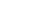from the horizontal as shown above. The angle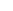is called as the angle of repose. The lateral pressure form the material is resisted by the side walls. The bunker floor takes up the total load of the material. The theory used in determination of lateral pressure in bunkers is Rankine 's Theory.

## Design Consideration of Bunkers

### 1. Design of Bunkers with Rectangular or Square Bottom

The main structural elements that constitutes a bunker are shown in figure-2. They comprise of
• Vertical walls
• Hopper Bottom
• Edge Beam (At the top level)
• Supporting ColumnsFig.2: Structural Elements of a bunker

The design Procedure can be explained in following steps:

### Step 1: Design of Vertical Walls

Based on Rankine's Theory, the lateral pressure applied on the vertical wall can be given by the formula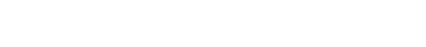Where, Pa = Lateral pressure intensity that is acting at a height of 'h'. L = Length of the bunker B = Breadth of the bunker a = Angle of surcharge (The material slope as shown in figure-3)= Angle of rupture w = density of the material stored in the bunkerFig.3: Representation of angle of surcharge (?) and pressure component acting on walls (p).

This pressure pa is acting in the direction parallel to angle of surcharge. So, the pressure that is applied on the vertical walls are the horizontal component of pa. Let it be p as shown in figure-3.#### Design Moments:

a) Negative Moments at the supports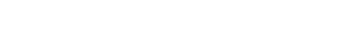b) Positive Bending Moment at the center of longer sides (AB or CD)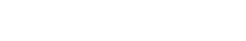c) Positive Bending Moment at the center of shorter sides (BC or AD)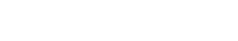#### Direct Tension:

a) Tension in long walls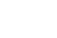b) Tension in Short walls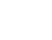#### Effective depth:

The effective depth is given by the formulaTo resist maximum bending moment adequate thickness should be provided. The reinforcement details are provided for the vertical walls based on the maximum bending moments and the direct tension design values. The reinforcement obtained from above equation (Ast), is arranged in the horizontal direction. Minimum distribution reinforcement is provided in the vertical direction. Minimum cross section of 300mm x 300mm edge beams are provided at the top, to facilitate attachments used by conveyor supports.

### Step 2: Design of Hopper Bottom

The hopper bottom is designed for direct tension caused due to: a) Self weight of the material b) Self weight of sloping slabFig. 4: Sloping slab in the hopper subjected to direct tensionFig.5: Sloping Slab in Hopper Bottom Subjected to bending

From figure-4 and 5, wt = weight of material Calculation of Direct tension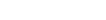Where,is the angle between the horizontal and the sloping slab.

### Calculation for Bending Moment

To determine the maximum moments at the supports and the center of the sloping slab, we need to determine the normal pressure intensity which is the sum of normal pressure due to material weight and the self-weight of the slab

#### a) Due to material weight

If w = density of the material h= average height at the center of the slope of bottom L = Effective span at the center of the slope, as shown in figure-5 Then, Normal pressure intensity for depth h is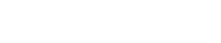hence after rearranging,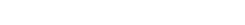#### b) Due to self-weight of slab

Let Wd be the self-weight of slab Its normal component with respect to plane of slab is given by,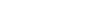Hence total normal pressure intensity is given by,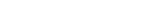Hence, Maximum Negative Bending Moment at Supports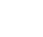Positive Bending Moment at the Center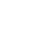### 2. Design consideration of Bunkers with Circular Bottom

For design of bunkers with circular cross section, vertical walls are subjected to a hoop tension along the diameter of the bunker. The value of hoop tension is given by the formula

Th = 0.5ph .D

D = Diameter of the bunker ph = horizontal component of pressure at a depth h from the top The reinforcement details are provided to resist the hoop tension for this a minimum thickness of 120mm is recommended. The hopper bottom is designed for both direct and hoop tension due to normal pressure on the sloping slabs. Minimum vertical reinforcement is provided based on the bar used.

### Step 3: Design of Columns

Columns are designed for compression and bending. The loads on the columns are due to: a) Vertical loads = weight of stored material + self-weight of members b) Horizontal loads = Wind Loads